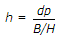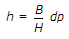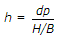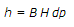# Civil Engineering - Elements of Remote Sensing

### Exercise :: Elements of Remote Sensing - Section 2

6.

Which one of the following statement is incorrect regarding the electromagnetic radiation?

 A. These are produced by the motion of electric charge B. The oscillation of charged particles sets up changing electric fields. C. The changing electric fields induce the changing magnetic fields in the surrounding medium. D. None of these

Explanation:

No answer description available for this question. Let us discuss.

7.

Consider the following statements regarding the satellite imaging :
1. The satellite orbit is fixed in the inertial space
2. During successive across-track imaging, the earth rotates beneath the sensor
3. The satellite images a skewed area
Which one of the following statements is/are correct ?

 A. 1, 2, 3 B. 1, 3 C. 2, 3 D. 1, 2

Explanation:

No answer description available for this question. Let us discuss.

8.

Which one of the following statements is correct?

 A. π radians equal to 180°. B. The cone subtended by an area on the sphere at the centre, is called the solid angle. C. The solid angle is equal to the ratio of the area on the sphere and the square of the radius of the sphere. D. The unit of the solid angle is steradian (Sr) E. All of these

Explanation:

No answer description available for this question. Let us discuss.

9.

If flying height of a spacecraft is H, the length of air base is B and the parallax difference between two points is dp, then the difference in height

 A.B.C.D.Explanation:

No answer description available for this question. Let us discuss.

10.

In GPS, receivers are used are ;

 A. electronic clocks B. atomic clocks C. quartz clocks D. mechanical clocks